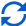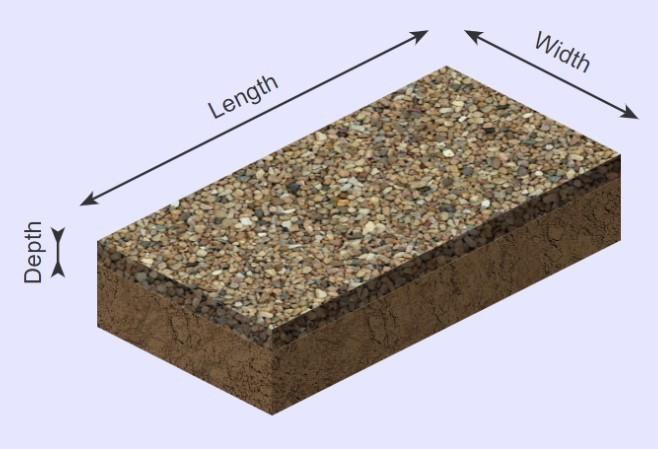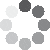Enter parameters LENGTH, WIDTH & DEPTH
Enter parameters
LENGTH, WIDTH & DEPTH
Length:
ft
• inches (in)
• feet (ft)
• yards (yd)
• centimeters (cm)
• meters (m)
Width:
ft
• inches (in)
• feet (ft)
• yards (yd)
• centimeters (cm)
• meters (m)
Depth:
in
• inches (in)
• feet (ft)
• yards (yd)
• centimeters (cm)
• meters (m)
Density:
89 - River rock lb/ft³
• River rock - 89 lb/ft³
• Custom
Price per unit of mass:
t
• pounds (lb)
• US short tons (t)
• imperial-long tons (long t)
• kilograms (kg)
Price per unit of volume:
yd³
• cubic yards (yd³)
• cubic feet (ft³)
• cubic inches (in³)
• cubic centimeters (cm³)
• cubic meters ()Result
Area:
0
ft²
• square feet (ft²)
• square inches (in²)
• square yards (yd²)
• square miles (mi²)
• acres (ac)
• square centimeters (cm²)
• square meters ()
Volume:
0
yd³
• cubic yards (yd³)
• cubic feet (ft³)
• cubic inches (in³)
• cubic centimeters (cm³)
• cubic meters ()
Weight:
0
t
• pounds (lb)
• US short tons(t)
• imperial-long tons (long t)
• kilograms (kg)
Cost:
$Enter parameters AREA & DEPTH Area: ft² • square feet (ft²) • square inches (in²) • square yards (yd²) • square miles (mi²) • acres (ac) • square centimeters (cm²) • square meters () Depth: in • inches (in) • feet (ft) • yards (yd) • centimeters (cm) • meters (m) Density: 89 - River rock lb/ft³ • River rock - 89 lb/ft³ • Custom Price per unit of mass: t • pounds (lb) • US short tons (t) • imperial-long tons (long t) • kilograms (kg) Price per unit of volume: yd³ • cubic yards (yd³) • cubic feet (ft³) • cubic inches (in³) • cubic centimeters (cm³) • cubic meters ()Result Volume: 0 yd³ • cubic yards (yd³) • cubic feet (ft³) • cubic inches (in³) • cubic centimeters (cm³) • cubic meters () Weight: 0 t • pounds (lb) • US short tons (t) • imperial-long tons (long t) • kilograms (kg) Cost:$
Enter parameters
VOLUME
Volume:
yd³
• cubic yards (yd³)
• cubic feet (ft³)
• cubic inches (in³)
• cubic centimeters (cm³)
• cubic meters ()
Density:
89 - River rock lb/ft³
• River rock - 89 lb/ft³
• Custom
Price per unit of mass:
t
• pounds (lb)
• US short tons (t)
• imperial-long tons (long t)
• kilograms (kg)
Price per unit of volume:
yd³
• cubic yards (yd³)
• cubic feet (ft³)
• cubic inches (in³)
• cubic centimeters (cm³)
• cubic meters ()Result
Weight:
0
t
• pounds (lb)
• US short tons (t)
• imperial-long tons (long t)
• kilograms (kg)
Cost:
$(8 votes, average: 4.63 out of 5)Loading... Similar Calculators: Real-time graphics. Make the calculations and see the changes. Real-time graphics. Make the calculations and see the changes. Real-time graphics. Make the calculations and see the changes. Embed Similar Calculators:Before you start any outdoor landscaping project, having an idea of how much material you will need is vital. We think that you’ll agree that being accurate in your estimations will save you both money and time. Our river rock calculator can help! 👍 Below, we will show you how to use the calculator to work out how much river rock you will need. We will detail the formulae used and give several full examples. Contents: ## River rock calculator formulaOnce you have entered the length, width and depth of the rock, the calculator will first work out the area in square feet: $$Area = Length \times Width$$ and the volume in cubic feet: $$Volume = Area \times Depth$$ In addition, if you know the density of the river rock, it can calculate the total weight: $$Weight = Density\,of\,River\,Rock × Volume$$ NB: The standard density of river rock is 89 lb/ft³. But you’re probably wondering: What if I don’t know the density of the rock? Well, you can easily calculate this if you know the mass and volume: $$Density = {Mass \over Volume}$$ ### How do you find the cost of the project? The calculator can use the price per unit mass/volume of the river rock to calculate the total cost of the material: $$Cost = Price\,Per\,Unit\,Mass × Weight$$ or $$Cost = Price\,Per\,Unit\,Volume × Volume$$ Of course, you may not be sure of the unit price. If so, then you can calculate this using the mathematical formula: $$Price\,Per\,Unit\,Mass = {Total\,Cost\,of\,River\,Rock \over Weight\,of\,River\,Rock}$$ or $$Price\,Per\,Unit\,Volume = {Total\,Cost\,of\,River\,Rock \over Volume\,of\,River\,Rock}$$ ### What if my measurements aren’t just in feet and inches? Our calculator is able to work with many units and does the appropriate conversions for you. Simply select the desired units, and the calculator will do the following conversions: $$1\,foot = 12\,inches = 0.33\,yards = 30.48\,centimeters = 0.3048\,meters$$ $$1\,US\,short\,ton = 2000\,pounds = 0.893\,imperial\,long\,ton = 907\,kg$$ Confused? 😕 Below, we provide three full examples detailing how the calculator finds the total volume, weight and cost. Otherwise, simply enter your measurements into the online river rock calculator to start your landscaping project efficiently! ## Example calculations • Calculate volume, weight and cost from length, width and depth Let’s say that I have an area of standard river rock (89 lb/ft³) measuring 6 feet in length and 4 feet in width. I want to ensure an equal coverage to a depth of 10 inches. The rock costs$60 per US short ton.

I want to calculate the volume, weight and cost of this landscaping project.

I would enter these measurements into the estimator which would perform the following operations:

$$Area = Length \times Width = 6\,ft × 4\,ft = 24\,ft^2$$

$$Volume = Area \times Depth = 24\,ft^2 × 10\,in = 20\,ft^3$$

$$Weight = Density\,of\,River\,Rock × Volume = 89\,lb/ft^3 × 20\,ft^3 = 0.89\,t$$

$$Cost = Price\,Per\,Unit\,Mass × Weight = 60\,/t × 0.89\,t = 53.4$$

• Using a known area and depth with custom aggregate

Let’s now say that I need to cover an area with a custom aggregate stone.

The square footage of the area is . I want to ensure an equal coverage to a depth of 2 yards.

I am unsure of the stone’s density, but 10 cubic feet weigh 700 pounds and cost $70 per US short ton. Therefore I can calculate the stone’s density: $$Density = {Mass \over Volume} = {700\,lb \over 10\,ft^3} = 70\,lb/ft^3$$ I would then enter the above measurements into the online calculator to work out the volume, weight and total cost: $$Volume = Area \times Depth = 20\,ft^2 × 2\,yds = 120\,ft^3$$ $$Weight = Density\,of\,Aggregate × Volume = 70\,lb/ft^3 × 120\,ft^3 = 4.2\,t$$ $$Cost = Price\,Per\,Unit\,Mass × Weight = 70\,/t × 4.2\,t = 294$$ • Using a known volume and calculating an unknown price per unit volume For our final example we will show you how to calculate the price per unit volume of a given river rock: Let’s say that I need 30 cubic feet of standard river rock (89 ). I can purchase 5 cubic yards of river rock at a price of$450.

I can therefore calculate the price per unit volume:

$$Price\,Per\,Unit\,Volume = {Total\,Cost\,of\,River\,Rock \over Volume\,of\,River\,Rock} = {450 \over 5\,yd^3} = 90\,/yd^3$$

I then enter the measurements into the online river rock calculator to work out the weight of the rock:

$$Weight = Density\,of\,River\,Rock × Volume = 89\,lb/ft^3 × 30\,ft^3 = 1.335\,t$$

and the total cost of the landscape project:

$$Cost = Price\,Per\,Unit\,Volume × Volume = 90\,/yd^3 × 30\,ft^3 = 100$$

It’s that simple! 🙂

Embed this calculator on your site!Add live graphics
Copied to clipboard! Preview